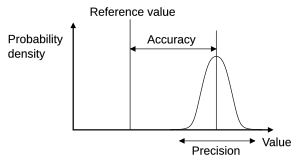# Accuracy and precision facts for kids

Kids Encyclopedia FactsAccuracy: how close results are to the true value
Precision: the repeatability of measurements

The accuracy and precision of measurements have special meanings in the fields of science, engineering, industry and statistics.

• The accuracy of a measurement system is how close it gets to a quantity's actual (true) value.
• The precision of a measurement system is the degree to which repeated measurements give the same results.

A measurement system can be accurate but not precise, precise but not accurate, neither, or both. For example, if an experiment contains a error in the way it is done, then increasing the sample size generally increases precision but does not improve accuracy. The end result would be a consistent, yet inaccurate, set of results from the flawed experiment. Eliminating the systematic error improves accuracy but does not change precision.

A measurement system is valid if it is both accurate and precise. Related terms include bias (non-random or directed effects caused by a factor or factors unrelated to the independent variable) and error (random variability).

## Related topics

The terminology is also applied to indirect measurements—that is, values obtained by a computational procedure from observed data.

In addition to accuracy and precision, measurements may also have a measurement resolution, which is the smallest change in the underlying physical quantity that produces a response in the measurement.

## Related pagesAccuracy and precision Facts for Kids. Kiddle Encyclopedia.